# Corresponding Angles

Corresponding Angles
Go back to  'Angles'

Hey kids, did you know that opening and shutting a lunchbox, solving a rubik's cube, and never ending parallel railway tracks are every day examples of corresponding angles?

You may have never realized the concepts you actually might be using in these cases. Have a look at the article to know what exactly you missed out!

## Lesson Plan

 1 Definition of Corresponding Angles 2 Important Notes on Corresponding Angles 3 Solved Examples on Corresponding Angles 4 Challenging Questions on Corresponding Angles 5 Interactive Questions on Corresponding Angles

## What Are Corresponding Angles?

Let's begin this topic by first understanding the meaning of corresponding angles.

The corresponding angles definition tells us that when two parallel lines are intersected by a third one, the angles that occupy the same relative position at each intersection are known to be corresponding angles to each other.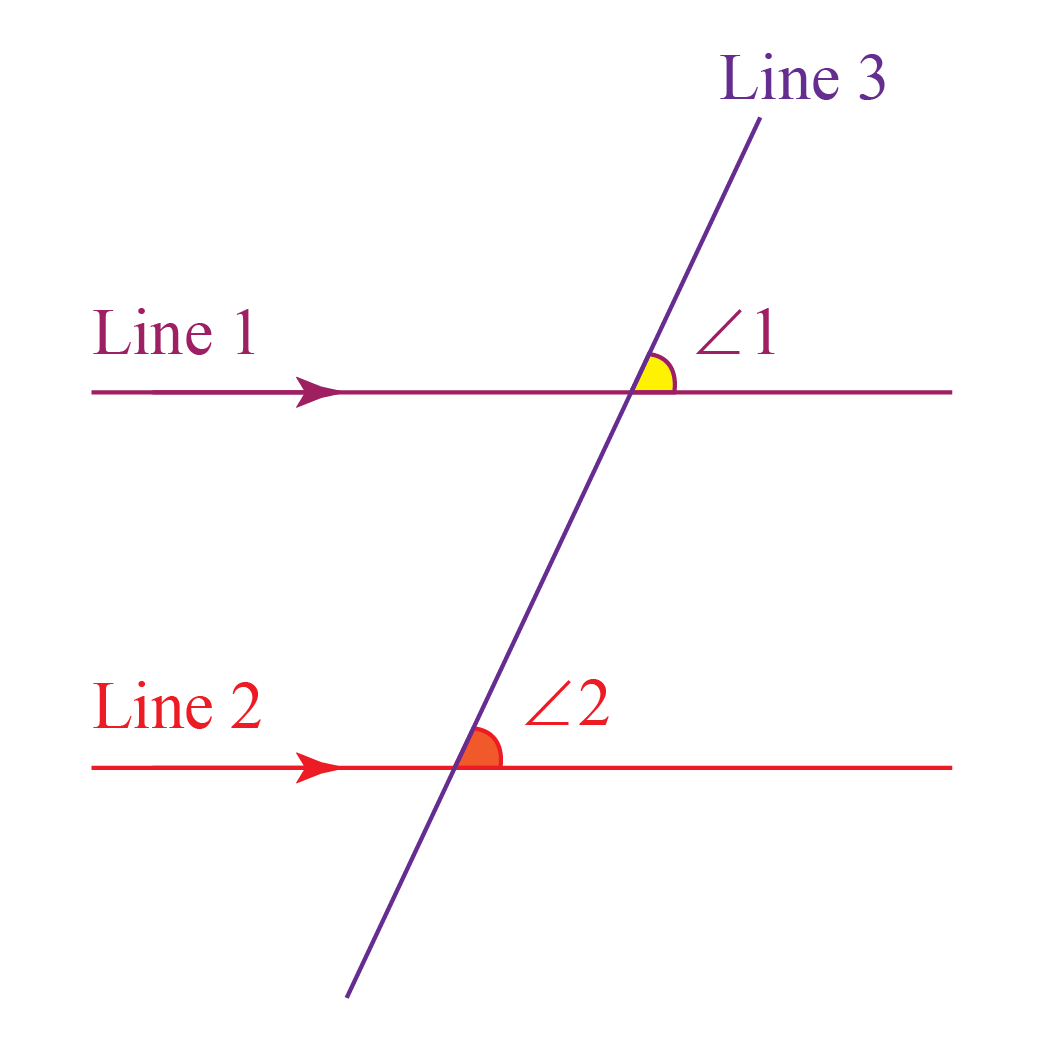Applying the Math definition for corresponding angles, we can see that:

• Lines 1 and 2 are parallel. Thus, we have two parallel lines

• Line 3 is intersecting lines 1 and 2. Thus, we have intersected parallel lines

• From the diagram, we can see that angles 1 and 2 are occupying the same relative position - the upper right side angles in the intersection region.

It is clear that our corresponding angles definition seems to be fulfilled.

Therefore, we can say that angles 1 and 2 are corresponding angles.

Now that we have understood the definition of corresponding angles, we can figure out whether any two given angles are corresponding or not in any given diagram.

Now, let us go a little deeper in to the meaning of corresponding angles.

The word “corresponding” itself suggests that the angles can be either analogous or equivalent (congruent).

Surprisingly, corresponding angles are considered to be analogous angles which are congruent.

Recalling our corresponding angles 1 and 2, we can tell that angles 1 and 2 are congruent.

## How do you find Corresponding Angles?

We know that each intersection point has 4 angles.

Now, each of the four angles in the first intersection region will have another one with the same relative position in the second intersection region.

Look at the simulation given here.

Click on any angle to know the other angle with the same relative position as the one you clicked.

Now, we will separate each of these four angles into different categories.

See the table below to get a better understanding of the different types of corresponding angles.

 Name of Angles Location Angles 1 and 5 Upper Right Side Angle Angles 2 and 6 Upper Left Side Angle Angles 3 and 7 Lower Right Side Angle Angles 4 and 8 Lower Left Side Angle

We already know that if a line intersects two parallel lines, then the corresponding angles in the two intersection regions are congruent.

But now, let's suppose that a line intersects two other lines (lines 1 and 2 that are not necessarily parallel).

Now the corresponding angles are found to be congruent.

Then, what can you say about the lines 1 and 2?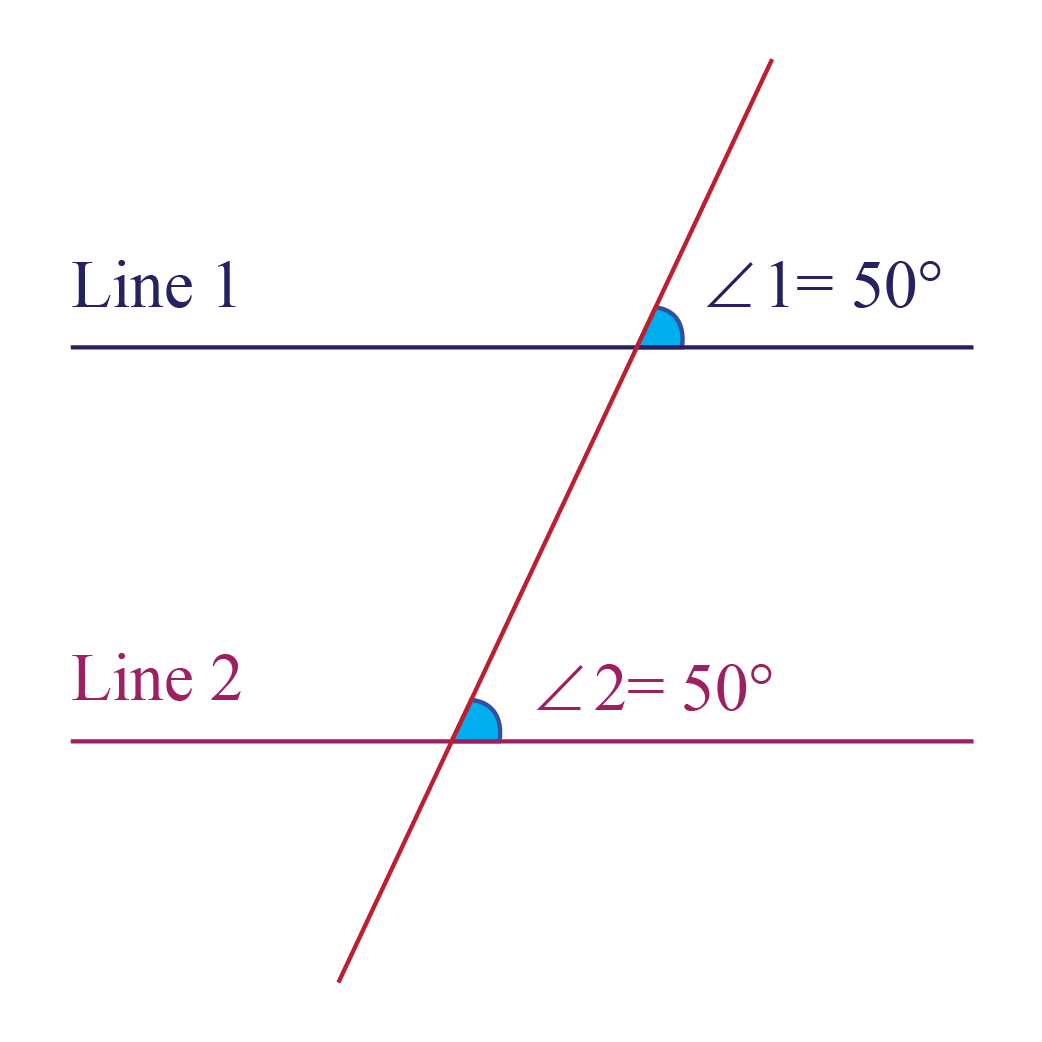Now, according to the corresponding angles theorem, the statement “If a line intersects two parallel lines, then the corresponding angles in the two intersection regions are congruent” is true either way.

Thus, the corresponding angles converse theorem would be, “If the corresponding angles in the two intersection regions are congruent, then the two lines are said to be parallel.”Important Notes
1. When two parallel lines are intersected by a third one, the angles that occupy the same relative position at each intersection are called corresponding angles to each other.
2. If the corresponding angles in the two intersection regions are congruent, then the two lines are said to be parallel.
3. The angles formed by corresponding angles are congruent.

## Solved Examples

There might have been a lot of real life situations where you may have used the concept of corresponding angles, or seen them without realising it.

Let us discuss some of the corresponding angles examples here.

 Example 1

Have you ever noticed a tall building?

In most tall buildings, each of its floors is designed in exactly the same way, especially the walls of the house on each floor.

Compare the corresponding angles in such a case.

Solution

Let us consider the bottom tiles of floor 1 as line 1 and that of floor 2 as line 2

Now, we know that line 3 is intersecting lines 1 and 2

In this figure, you can notice the geometry of the corresponding angles.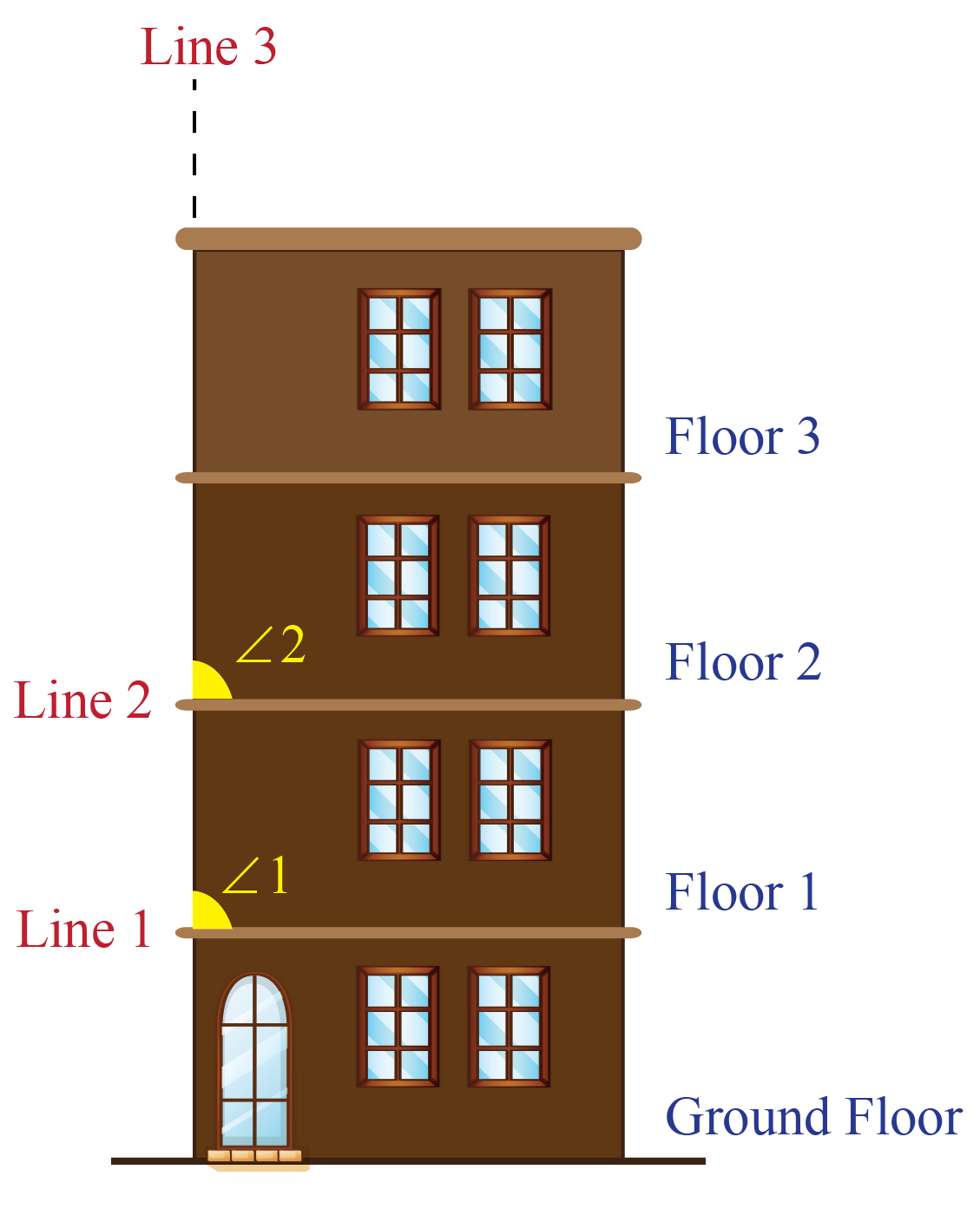Can you see any similarity between angles 1 and 2?

You can see that angles 1 and 2 are corresponding angles.

Not only that, as all the floors are always built parallel to each other, we can say that lines 1 and 2 are parallel.

 $$\therefore\; \angle1$$ is corresponding to $$\angle2$$
 Example 2

Did you ever have a parallelogram-shaped lunchbox in school?

How did you close this tiffin box?

You tried to find the best match of angles on the lid to close the box. Is that right?

Can you think of any reason why you did that?

Solution

The reason you did that was because you tried to find the best fit of congruent angles for closing the lid of the box.

As we know that corresponding angles are congruent, you tried to find the angles on the lid that best matched every corner’s corresponding angles in the box.

You were observing the geometry of the corresponding angles without realizing it.

##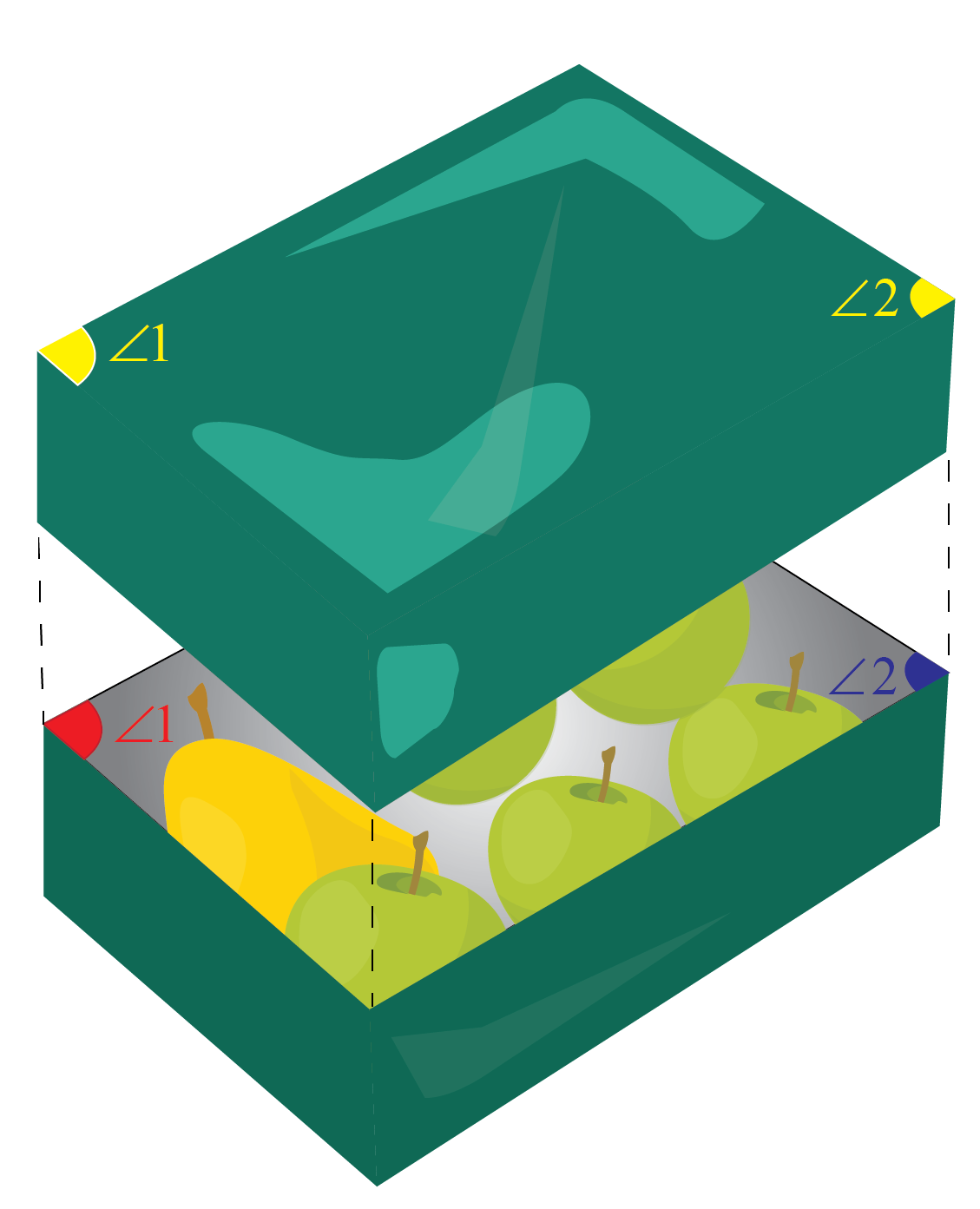$$\therefore$$ The angles in a tiffin box are corresponding.
 Example 3

Did you ever notice the parallel lines on a railway track?

There are multiple intersections of different smaller lines with the two main parallel track lines.

Compare the angles made by the intersection.

Solution

Can you see any similarity between the concept of congruent angles and angles 1 and 2 in the diagram given below?

Recall the definition we used for corresponding angles to fit into our angles shown here.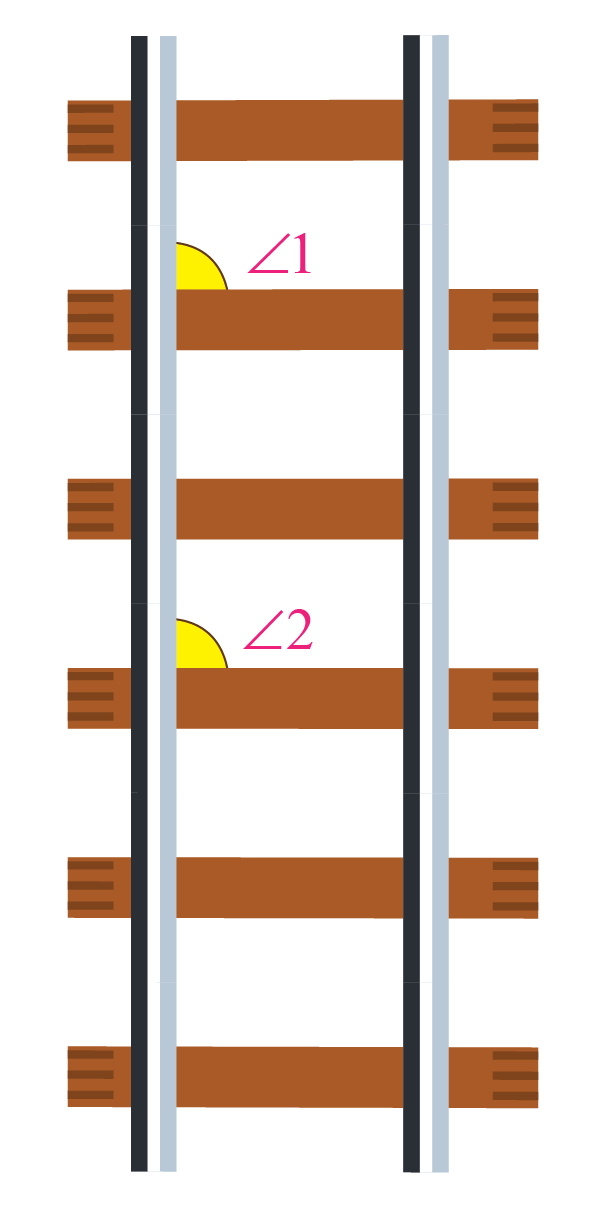You will be able to see that if we consider the track lines to be parallel, angles 1 and 2 can be considered as corresponding angles.

This is according to the corresponding angles in Math definition.

 $$\therefore\; \angle1$$ is corresponding to $$\angle2$$
 Example 4

Did you ever notice a Rubik's cube?

We know that all the lines are parallel and all the angles are congruent.

Now, can you think of any reason why it is so?

Solution

This is because there are parallel lines, and these lines contain multiple corresponding angles.

Some of it can be seen here.

Try finding out other corresponding angles as well.

##Can you see any similarity between the angles that are marked?

You can see that the angles are corresponding angles.

Not only that, as all the lines are always built parallel to each other in a Rubik's cube, we can say that the angles will always be corresponding.

 $$\therefore$$ The marked angles are corresponding angles.
 Example 5

Have you ever come across two parallel streets?

There is usually a connecting road between the two streets that also intersects it.

Now, try to relate the angles made by the street at each intersection point with the two parallel roads.

Solution

Apply our definition for corresponding angles to the angles shown here.

You will see that according to our definition, these angles are corresponding!

##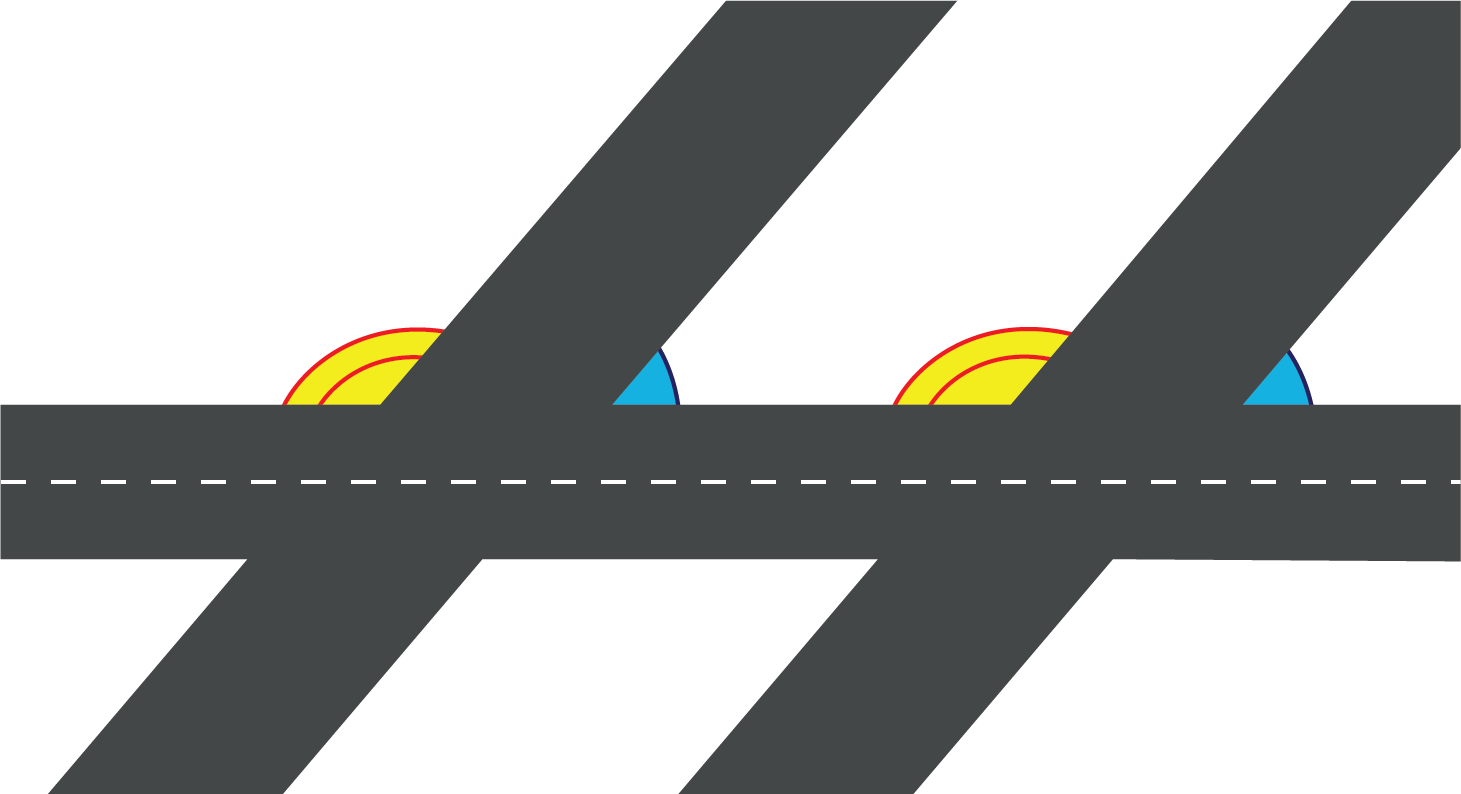Not only that, as all the streets are always built parallel to each other, we can also say that angles residing on the same relative positions on the streets will always be corresponding angles.

 $$\therefore$$  Angles formed by parallel streets are corresponding angles.Challenging Questions

The following information has been given regarding angles A, B, C and D :

1. A and B are corresponding angles
2. B and C are supplementary angles
3. C and D are co - interior angles

Find the angle (other than B), which will be congruent to angle A.

## Interactive Questions

Here are a few activities for you to practice.

## Let's Summarize

The mini-lesson targeted the fascinating concept of Is 51 a Prime number. The math journey around Is 51 a Prime number starts with what a student already knows, and goes on to creatively crafting a fresh concept in the young minds. Done in a way that not only it is relatable and easy to grasp, but also will stay with them forever. Here lies the magic with Cuemath.

At Cuemath, our team of math experts is dedicated to making learning fun for our favorite readers, the students!

Through an interactive and engaging learning-teaching-learning approach, the teachers explore all angles of a topic.

Be it worksheets, online classes, doubt sessions, or any other form of relation, it’s the logical thinking and smart learning approach that we, at Cuemath, believe in.

## 1. Do corresponding angles add up to 180?

Yes, corresponding angles can add up to 180. In some cases when both angles are $$90^o$$ each, the sum will be $$180^o$$.

## 2. Alternate and corresponding angles

Alternate angles are angles that are at relatively opposite positions to each other; while the corresponding angles are the angles that are at relatively same positions to each other.

## 3. Consecutive interior angles

The consecutive interior angles are the angles that are on the same side of the transversal, but inside the two parallel lines.

Geometry
grade 9 | Questions Set 1
Geometry
Geometry
grade 9 | Questions Set 2
Geometry